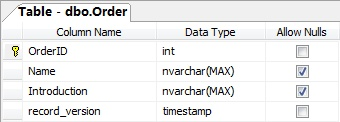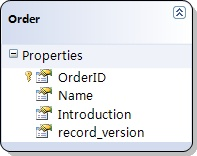## 在Linq to Sql中管理并发更新时的冲突（3）：使用记录的时间戳进行检测

2007-11-23 09:21 by Jeffrey Zhao, ... 阅读, ... 评论, 收藏, 编辑try{    LinqToSqlDemoDataContext dataContext = new LinqToSqlDemoDataContext();    Order order = dataContext.Orders.Single(o => o.OrderID == 1);    order.Name = "New Order Name";    dataContext.Log = Console.Out;    // 在下面的语句上设置一个断点    dataContext.SubmitChanges();}catch (ChangeConflictException e){    Console.WriteLine(e.Message);}Console.ReadLine();

UPDATE [dbo].[Order]SET [Name] = @p2WHERE ([OrderID] = @p0) AND ([record_version] = @p1)SELECT [t1].[record_version]FROM [dbo].[Order] AS [t1]WHERE ((@@ROWCOUNT) > 0) AND ([t1].[OrderID] = @p3)-- @p0: Input Int (Size = 0; Prec = 0; Scale = 0) -- @p1: Input Timestamp (Size = 8; Prec = 0; Scale = 0) [SqlBinary(8)]-- @p2: Input NVarChar (Size = 14; Prec = 0; Scale = 0) [New Order Name]-- @p3: Input Int (Size = 0; Prec = 0; Scale = 0) -- Context: SqlProvider(Sql2005) Model: AttributedMetaModel Build: 3.5.21022.8Row not found or changed.

try{    LinqToSqlDemoDataContext dataContext = new LinqToSqlDemoDataContext();    Order order1 = dataContext.Orders.Single(o => o.OrderID == 1);    Order order2 = dataContext.Orders.Single(o => o.OrderID == 2);    Order order3 = dataContext.Orders.Single(o => o.OrderID == 3);    Console.WriteLine('Order 1: ' + order1.Introduction);    Console.WriteLine('Order 2: ' + order2.Introduction);    Console.WriteLine('Order 3: ' + order3.Introduction);    Console.WriteLine();    order1.Introduction = 'Order 1 modified.';    order2.Introduction = 'Order 2 modified.';    order3.Introduction = 'Order 3 modified.';    dataContext.Log = Console.Out;    // 在下面的语句上设置一个断点    dataContext.SubmitChanges();}catch (ChangeConflictException e){    Console.WriteLine('---------- ' + e.Message + ' ----------');}LinqToSqlDemoDataContext db = new LinqToSqlDemoDataContext();Order o1 = db.Orders.Single(o => o.OrderID == 1);Order o2 = db.Orders.Single(o => o.OrderID == 2);Order o3 = db.Orders.Single(o => o.OrderID == 3);Console.WriteLine('Order 1: ' + o1.Introduction);Console.WriteLine('Order 2: ' + o2.Introduction);Console.WriteLine('Order 3: ' + o3.Introduction);Console.ReadLine();

 OrderID Name Introduction record_version 1 Order 1 This is order 1 2 Order 2 This is order 2 3 Order 3 This is order 3

UPDATE Order SET OrderID = "New Order 2" WHERE OrderID = 2

Order 1: This is order 1Order 2: This is order 2Order 3: This is order 3UPDATE [dbo].[Order]SET [Introduction] = @p2WHERE ([OrderID] = @p0) AND ([record_version] = @p1)SELECT [t1].[record_version]FROM [dbo].[Order] AS [t1]WHERE ((@@ROWCOUNT) > 0) AND ([t1].[OrderID] = @p3)-- @p0: Input Int (Size = 0; Prec = 0; Scale = 0) -- @p1: Input Timestamp (Size = 8; Prec = 0; Scale = 0) [SqlBinary(8)]-- @p2: Input NVarChar (Size = 26; Prec = 0; Scale = 0) [Order 1 modified.]-- @p3: Input Int (Size = 0; Prec = 0; Scale = 0) -- Context: SqlProvider(Sql2005) Model: AttributedMetaModel Build: 3.5.21022.8UPDATE [dbo].[Order]SET [Introduction] = @p2WHERE ([OrderID] = @p0) AND ([record_version] = @p1)SELECT [t1].[record_version]FROM [dbo].[Order] AS [t1]WHERE ((@@ROWCOUNT) > 0) AND ([t1].[OrderID] = @p3)-- @p0: Input Int (Size = 0; Prec = 0; Scale = 0) -- @p1: Input Timestamp (Size = 8; Prec = 0; Scale = 0) [SqlBinary(8)]-- @p2: Input NVarChar (Size = 26; Prec = 0; Scale = 0) [Order 2 modified.]-- @p3: Input Int (Size = 0; Prec = 0; Scale = 0) -- Context: SqlProvider(Sql2005) Model: AttributedMetaModel Build: 3.5.21022.8---------- 1 of 2 updates failed. ----------Order 1: This is order 1Order 2: This is order 2Order 3: This is order 3